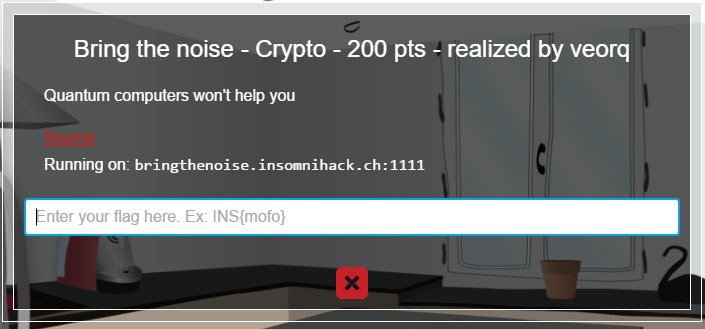# Insomnihack Teaser 2016 - Bring the noise - Crypto - 200 pts

## January 17, 2016

Reading time ~2 minutesGreat challenge! Connecting to the server seen we’re given a challenge. This is a standard challenge/response scenario designed just to prevent rapid brute force attacks against the server. We solve it quickly with itertools and move forward to the real problem.

Past the initial challenge response, we’re given set of 40 lists of seven integers per list. Upon inspection of the server source code which we’re also given, we see that what we have is a list of 40 coefficients and the result of a multiplication of those coefficients (mod 8) and a randomly chosen single solution. Additionally a “vibration” is factored in in such a way that it can vary the result +/- 1.

My solution was to simply split the coefficients in the input and iterate (again, thanks itertools!) through all possible solutions searching for a solution that best fits the value in the results field (give or take 1). The math we can re-use straight from the server code so very little hard stuff to do here.

A good fit is anything that met > 30 of the 40 equations given to us by the server. This seemed to be good enough because my solution worked each time I tried it.

Source code below:

``````#!/usr/bin/python

import os
import itertools

from Crypto.Hash import MD5

from pwn import *

HOST = 'bringthenoise.insomnihack.ch'
PORT = 1111

POWLEN = 5

conn = remote(HOST, PORT)
challenge = conn.recvline().split()
print "[*] Got challenge: " + challenge

responsehash = ""
charset = "abcdefghijklmnopqrstuvwxyz0123456789"

for i in itertools.product(charset, repeat=5):
responsehash = MD5.new(''.join(i)).hexdigest()

if responsehash[:POWLEN] == challenge:
response = ''.join(i)
break

print "[*] Sending challenge response: " + response
conn.sendline(response)

puzzle = conn.recvlines(40)

print "[*] Received equation sets. Computing candidate solutions..."

def checkmath(attempt, equation, result):
check = sum([attempt[i]*equation[i] for i in range(6)]) % 8

if check == result or check == (result + 1) or check == (result - 1):
return True
else:
return False

for k in itertools.product(range(8),repeat=6):
truthlist = []
for p in puzzle:
coefs  = map(int, p.split(",")[:6])
result = int(p.split(","))

if checkmath(k,coefs,result):
truthlist.append(1)

if len(truthlist) > 30:
print "[+] " + repr(k) + " Satisfied " + str(len(truthlist)) + " equations"
solution = map(str, k)
conn.recvline()
conn.sendline(", ".join(solution))
flag = conn.recvline()
print "[+] Got flag: " + flag
``````

Which, when run, results in the flag below:

``````root@ubuntu:~/insomnihack/bringnoise# ./solve.py
[+] Opening connection to bringthenoise.insomnihack.ch on port 1111: Done
[*] Got challenge: 2f58a
[*] Sending challenge response: a505f
[*] Received equation sets. Computing candidate solutions...
[+] (, 2, 7, 2, 7, 3) Satisfied 33 equations
[+] Got flag: INS{ErrorsOccurMistakesAreMade}
``````

Thanks for reading my writeups!

### niteCTF - CBC-Jail

A unique combination of Python jailbreak and crypto flaw that had me learning a lot about AES-CBC mode. Super fun for me to get this solu...… Continue reading

#### niteCTF - Rabin to the Rescue

Published on December 12, 2021

#### HTB CyberSanta 2021 - Crypto Writeups

Published on December 04, 2021